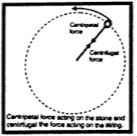Define the centripetal force. Derive the relation of centripetal force on a body moving in a circle?

Difficulty: Easy

Centripetal Force:

Centripetal force is a force that keeps a body moving in a circle. The center-seeking force is called the centripetal force. It keeps the body moving in a circle. Centripetal force always acts perpendicular to the motion of the body.

Examples:

Let us study the centripetal forces in the following examples:

• A stone tied to one end of a string rotating in a circle. The tension in the string provides the necessary centripetal force. It keeps the stone remaining in the circle. If the string is not strong enough to provide the necessary tension, it breaks and the stone moves away along a tangent to the circle
• The moon revolves around the Earth. The gravitational force of the Earth provides the necessary centripetal force.

Derivation of centripetal Force:

Let a body of mass m moves with uniform speed v in a circle of radius r. The acceleration ac produced by the centripetal force Fc is given by$Centripetal \: acceleration\: a_{c}=\frac{v^{2}}{r}$ .....(i)

According to Newton's second law of motion, the centripetal force Fc is given by

$F_{c}=m\: a_{c}$......(ii)

$F_{c}=\frac{mv^{2}}{r}$.....(iii)

Factors on which centripetal force depends:

Equation $F_{c}=\frac{mv^{2}}{r}$ shows that the centripetal force needed by a body moving in a circle depends on:

1. The mass m of the body
2. Square of its velocity v
3. Reciprocal to the radius r of the circle.